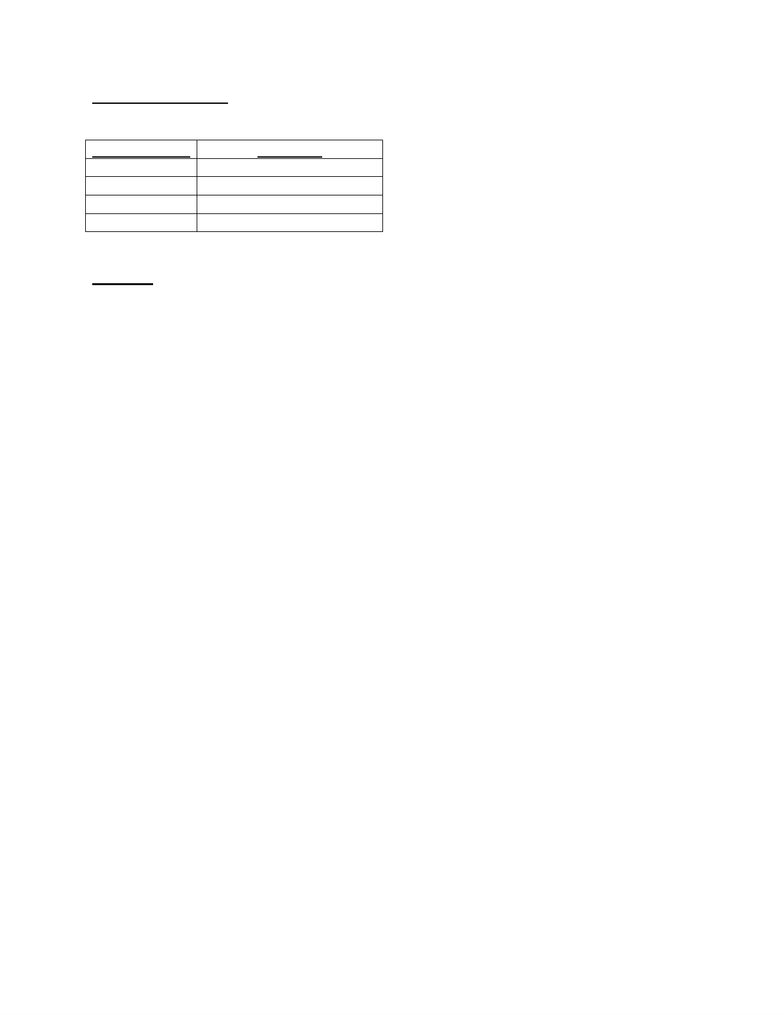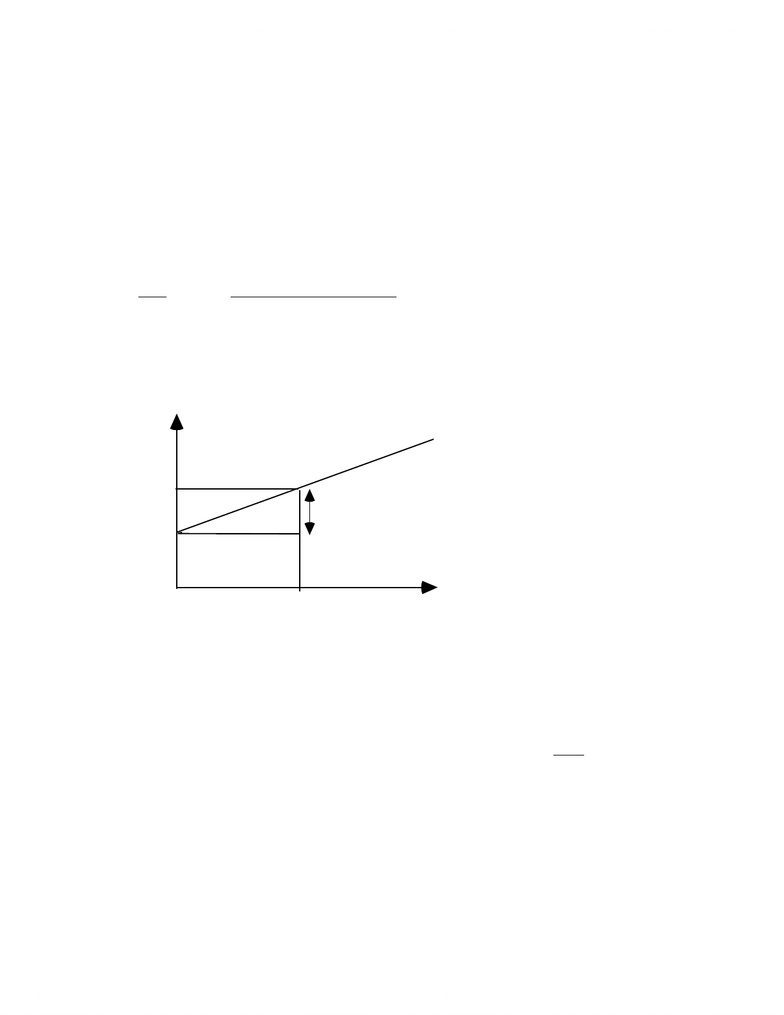Class Notes (1,100,000)
CA (620,000)
York (40,000)
Lecture

ADMS 3530 Lecture Notes - Money Market Fund, Risk Premium, Chc Helicopter

Department
Course Code
Professor
Lois King

This preview shows pages 1-2. to view the full 7 pages of the document.Review Questions 10
Textbook Page
Questions
358
2, 3, 4, 5
361
12, 14, 16, 17, 20
362
21. 28, 29, 30
363
31, 32, 33, 34, 35
Solutions
2. The risks of deaths of individual policyholders are largely independent, and therefore are
diversifiable. Therefore, the insurance company is satisfied to charge a premium that
reflects actuarial probabilities of death, without an additional risk premium. In contrast,
flood damage is not independent across policyholders. If my coastal home floods in a
storm, there is a greater chance that my neighbor's will too. Because flood risk is not
diversifiable, the insurance company may not be satisfied to charge a premium that reflects
only the expected value of payouts.
3. The actual returns on the Snake Oil fund exhibit considerable variation around the
regression line. This indicates that the fund is subject to diversifiable risk: it is not well
diversified. The variation in the fund's returns is influenced by more than just market-wide
events.
4. Investors would buy shares of firms with high degrees of diversifiable risk, and earn high-
risk premiums. However, by holding these shares in diversified portfolios, they would not
necessarily bear a high degree of portfolio risk. This would represent a profit opportunity,
however. As investors seek these shares, we would expect their prices to rise, and the
expected rate of return to investors buying at these higher prices to fall. This process would
continue until the reward for bearing diversifiable risk dissipated.
5. a. Required return = rf + (rm rf) = 4% + .6 (11% 4%) = 8.2%
With an IRR of 14%, the project is attractive.
b. If beta = 1.6, required return increases to:
4% + 1.6 (11% 4%) = 15.2%
which is greater than the project IRR. You should now reject the project.
c. Given its IRR, the project is attractive when its risk and therefore its required return
are low. At a higher risk level, the IRR is no longer higher than the expected return on
comparable risk assets available elsewhere in the capital market.

Only pages 1-2 are available for preview. Some parts have been intentionally blurred.- 2 -
12. Figure follows below.
Cost of capital = risk-free rate + beta × market risk premium
Since the risk-free rate is 4% and the market risk premium is 7%, we can write the cost of
capital as:
Cost of capital = 4% + beta × 7%
Cost of capital (from CAPM)
Beta = 10% + beta × 8%
.75 4% + .75 7% = 9.25%
1.75 4% + 1.75 7% = 16.25%
beta
r
1.0
4%
11%
7% = market risk
SML
0
The cost of capital of each project is calculated using the above CAPM formula. Thus, for
Project P, its cost of capital is: 4% + 1.0 × 7% = 11%.
If the cost of capital is greater than IRR, then the NPV is negative. If the cost of capital
equals the IRR, then the NPV is zero. Otherwise, if the cost of capital is less than the IRR,
the NPV is positive.
Project
Beta
Cost of capital
IRR
NPV
P
1.0
11.0%
11%
0
Q
0.0
4.0
6
+
R
2.0
18.0
17
S
0.4
6.8
7
+
T
1.6
15.2
16
+
You're Reading a Preview

Unlock to view full version